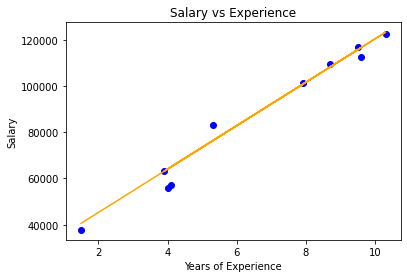Skip to content
Related Articles
Implementation of Lasso Regression From Scratch using Python
• Last Updated : 05 Sep, 2020

#### Prerequisites:

1. Linear Regression
2. Gradient Descent

#### Introduction:

Lasso Regression is also another linear model derived from Linear Regression which shares the same hypothetical function for prediction. The cost function of Linear Regression is represented by J.Here, m is the total number of training examples in the dataset.
h(x(i)) represents the hypothetical function for prediction.
y(i) represents the value of target variable for ith training example.



Linear Regression model considers all the features equally relevant for prediction. When there are many features in the dataset and even some of them are not relevant for the predictive model. This makes the model more complex with a too inaccurate prediction on the test set ( or overfitting ). Such a model with high variance does not generalize on the new data. So, Lasso Regression comes for the rescue. It introduced an L1 penalty ( or equal to the absolute value of the magnitude of weights) in the cost function of Linear Regression. The modified cost function for Lasso Regression is given below.Here, w(j) represents the weight for jth feature.
n is the number of features in the dataset.
lambda is the regularization strength.



Lasso Regression performs both, variable selection and regularization too.

#### Mathematical Intuition:

During gradient descent optimization,  added l1 penalty shrunk weights close to zero or zero.  Those weights which are shrunken to zero eliminates the features present in the hypothetical function. Due to this, irrelevant features don’t participate in the predictive model. This penalization of weights makes the hypothesis more simple which encourages the sparsity ( model with few parameters ).

If the intercept is added, it remains unchanged.

We can control the strength of regularization by hyperparameter lambda. All weights are reduced by the same factor lambda.

Different cases for tuning values of lambda.

1. If lambda is set to be 0,   Lasso Regression equals Linear Regression.
2. If lambda is set to be infinity, all weights are shrunk to zero.

If we increase lambda, bias increases if we decrease the lambda variance increase. As lambda increases, more and more weights are shrunk to zero and eliminates features from the model.

#### Implementation

Dataset used in this implementation can be downloaded from the link.

It has 2 columns — “YearsExperience” and “Salary” for 30 employees in a company. So in this, we will train a Lasso Regression model to learn the correlation between the number of years of experience of each employee and their respective salary. Once the model is trained, we will be able to predict the salary of an employee on the basis of his years of experience.

Code:

 # Importing libraries  import numpy as np  import pandas as pd  from sklearn.model_selection import train_test_split  import matplotlib.pyplot as plt  # Lasso Regression  class LassoRegression() :          def __init__( self, learning_rate, iterations, l1_penality ) :                  self.learning_rate = learning_rate                  self.iterations = iterations                  self.l1_penality = l1_penality              # Function for model training                  def fit( self, X, Y ) :                  # no_of_training_examples, no_of_features                  self.m, self.n = X.shape                  # weight initialization                  self.W = np.zeros( self.n )                  self.b = 0                  self.X = X                  self.Y = Y                  # gradient descent learning                          for i in range( self.iterations ) :                          self.update_weights()                      return self          # Helper function to update weights in gradient descent          def update_weights( self ) :                     Y_pred = self.predict( self.X )                  # calculate gradients                    dW = np.zeros( self.n )                  for j in range( self.n ) :                          if self.W[j] > 0 :                                  dW[j] = ( - ( 2 * ( self.X[:, j] ).dot( self.Y - Y_pred ) )                                                     + self.l1_penality ) / self.m                      else :                                  dW[j] = ( - ( 2 * ( self.X[:, j] ).dot( self.Y - Y_pred ) )                                                     - self.l1_penality ) / self.m                 db = - 2 * np.sum( self.Y - Y_pred ) / self.m                   # update weights              self.W = self.W - self.learning_rate * dW              self.b = self.b - self.learning_rate * db                  return self          # Hypothetical function  h( x )           def predict( self, X ) :              return X.dot( self.W ) + self.b        def main() :          # Importing dataset          df = pd.read_csv( "salary_data.csv" )      X = df.iloc[:, :-1].values      Y = df.iloc[:, 1].values          # Splitting dataset into train and test set      X_train, X_test, Y_train, Y_test = train_test_split( X, Y, test_size = 1 / 3, random_state = 0 )          # Model training          model = LassoRegression( iterations = 1000, learning_rate = 0.01, l1_penality = 500 )      model.fit( X_train, Y_train )          # Prediction on test set      Y_pred = model.predict( X_test )          print( "Predicted values ", np.round( Y_pred[:3], 2 ) )           print( "Real values      ", Y_test[:3] )          print( "Trained W        ", round( model.W, 2 ) )          print( "Trained b        ", round( model.b, 2 ) )          # Visualization on test set           plt.scatter( X_test, Y_test, color = 'blue' )          plt.plot( X_test, Y_pred, color = 'orange' )          plt.title( 'Salary vs Experience' )          plt.xlabel( 'Years of Experience' )          plt.ylabel( 'Salary' )          plt.show()        if __name__ == "__main__" :           main()

Output:

Predicted values  [ 40600.91 123294.39  65033.07]
Real values       [ 37731 122391  57081]
Trained W         9396.99
Trained b         26505.43Visualization

Note: It automates certain parts of model selection and sometimes called variables eliminator.My Personal Notes arrow_drop_up